# PostgreSQL 9.5 对pgbench的功能增强。

• Allow counting of pgbench transactions that take over a specified amount of time (Fabien Coelho)

This is controlled by new --latency-limit option.

• Allow pgbench to generate Gaussian/exponential distributions using \setrandom (Kondo Mitsumasa, Fabien Coelho)

• Allow pgbench's \set command to handle multi-operator expressions (Robert Haas, Fabien Coelho)

## 1. 允许在\set中使用更复杂的表达式（正负，加 减 乘 除 余，括号）。

\set varname expression
Sets variable varname to an integer value calculated from expression. The expression may contain integer constants such as 5432, references to variables :variablename, and expressions composed of unary (-) or binary operators (+, -, *, /, %) with their usual associativity, and parentheses.

Examples:
\set ntellers 10 * :scale
\set aid (1021 * :ntellers) % (100000 * :scale) + 1

## 2. 允许设置--latency-limit，执行时间超过限制的SQL将分开统计和显示为late，与--rate同时使用时，超出限制并且未发送到数据库执行的SQL记为skipped。

-L limit
--latency-limit=limit
Transaction which last more than limit milliseconds are counted and reported separately, as late.

When throttling is used (--rate=...), transactions that lag behind schedule by more than limit ms, and thus have no hope of meeting the latency limit, are not sent to the server at all. They are counted and reported separately as skipped.

-R rate
--rate=rate
Execute transactions targeting the specified rate instead of running as fast as possible (the default). The rate is given in transactions per second. If the targeted rate is above the maximum possible rate, the rate limit won't impact the results.

The rate is targeted by starting transactions along a Poisson-distributed schedule time line. The expected start time schedule moves forward based on when the client first started, not when the previous transaction ended. That approach means that when transactions go past their original scheduled end time, it is possible for later ones to catch up again.

When throttling is active, the transaction latency reported at the end of the run is calculated from the scheduled start times, so it includes the time each transaction had to wait for the previous transaction to finish. The wait time is called the schedule lag time, and its average and maximum are also reported separately. The transaction latency with respect to the actual transaction start time, i.e. the time spent executing the transaction in the database, can be computed by subtracting the schedule lag time from the reported latency.

If --latency-limit is used together with --rate, a transaction can lag behind so much that it is already over the latency limit when the previous transaction ends, because the latency is calculated from the scheduled start time. Such transactions are not sent to the server, but are skipped altogether and counted separately.

A high schedule lag time is an indication that the system cannot process transactions at the specified rate, with the chosen number of clients and threads. When the average transaction execution time is longer than the scheduled interval between each transaction, each successive transaction will fall further behind, and the schedule lag time will keep increasing the longer the test run is. When that happens, you will have to reduce the specified transaction rate.

## 3. 可以生成正态分布或指数分布的测试数据。

(原来只能生成概率一致的随机分布值)

\setrandom varname min max [ uniform | { gaussian | exponential } threshold ]
Sets variable varname to a random integer value between the limits min and max inclusive. Each limit can be either an integer constant or a :variablename reference to a variable having an integer value.

67%的值分布在以min,max数学期望为中心的 1.0 / threshold 这个区间。
95%的值分布在min,max数学期望为中心的 2.0 / threshold 这个区间。

By default, or when uniform is specified, all values in the range are drawn with equal probability. Specifying gaussian or exponential options modifies this behavior; each requires a mandatory threshold which determines the precise shape of the distribution.

For a Gaussian distribution, the interval is mapped onto a standard normal distribution (the classical bell-shaped Gaussian curve) truncated at -threshold on the left and +threshold on the right.
To be precise, if PHI(x) is the cumulative distribution function of the standard normal distribution,
with mean mu defined as (max + min) / 2.0,
then value i between min and max inclusive is drawn with probability:
min,max区间取i值的概率如下，(其中PHI(x)是正态分布的积分函数)：
(PHI(2.0 * threshold * (i - min - mu + 0.5) / (max - min + 1)) - PHI(2.0 * threshold * (i - min - mu - 0.5) / (max - min + 1))) / (2.0 * PHI(threshold) - 1.0).
Intuitively, the larger the threshold, the more frequently values close to the middle of the interval are drawn, and the less frequently values close to the min and max bounds.
About 67% of values are drawn from the middle 1.0 / threshold and 95% in the middle 2.0 / threshold;
67%的值分布在以min,max数学期望为中心的 1.0 / threshold 这个区间。
95%的值分布在min,max数学期望为中心的 2.0 / threshold 这个区间。
for instance, if threshold is 4.0, 67% of values are drawn from the middle quarter and 95% from the middle half of the interval.
The minimum threshold is 2.0 for performance of the Box-Muller transform. (性能考虑，2.0为最小threshold值)。

For an exponential distribution, the threshold parameter controls the distribution by truncating a quickly-decreasing exponential distribution at threshold, and then projecting onto integers between the bounds.
To be precise, value i between min and max inclusive is drawn with probability:
min,max区间取i值的概率如下：
(exp(-threshold*(i-min)/(max+1-min)) - exp(-threshold*(i+1-min)/(max+1-min))) / (1.0 - exp(-threshold)).

Intuitively, the larger the threshold, the more frequently values close to min are accessed, and the less frequently values close to max are accessed. (threshold越大，随机值接近min的概率越大，反之，threshold越小，随机值接近max的概率越大)
The closer to 0 the threshold, the flatter (more uniform) the access distribution.

A crude approximation of the distribution is that the most frequent 1% values in the range, close to min, are drawn threshold% of the time.
The threshold value must be strictly positive.

Example:
\setrandom aid 1 :naccounts gaussian 5.0

### 生成高斯分布的一组数据。

postgres=# create table test(id int);
CREATE TABLE
postgres=# \q

pg95@db-172-16-3-150-> vi test.sql
\setrandom id 1 50000 gaussian 100.0
insert into test values (:id);

--latency-limit=0.2 表示响应时间超过0.2毫秒的请求另外显示。

pg95@db-172-16-3-150-> pgbench -M prepared -n -r -f ./test.sql -P 2 -c 16 -j 16 -T 10 --latency-limit=0.2
progress: 2.0 s, 93423.3 tps, lat 0.164 ms stddev 0.122
progress: 4.0 s, 95957.7 tps, lat 0.164 ms stddev 0.109
progress: 6.0 s, 95074.0 tps, lat 0.166 ms stddev 0.116
progress: 8.0 s, 95760.4 tps, lat 0.165 ms stddev 0.111
progress: 10.0 s, 95711.8 tps, lat 0.165 ms stddev 0.108
transaction type: Custom query
scaling factor: 1
query mode: prepared
number of clients: 16
duration: 10 s
number of transactions actually processed: 951872

number of transactions above the 0.2 ms latency limit: 175929 (18.482 %)
latency average: 0.165 ms
latency stddev: 0.113 ms
tps = 95165.492751 (including connections establishing)
tps = 95694.059134 (excluding connections establishing)
statement latencies in milliseconds:
-0.002321       \setrandom id 1 50000 gaussian 100.0
0.160266        insert into test values (:id);

> install.packages("RPostgreSQL")
> library(RPostgreSQL)

1: 程辑包‘RPostgreSQL’是用R版本3.1.3 来建造的
2: 程辑包‘DBI’是用R版本3.1.3 来建造的

> drv <- dbDriver("PostgreSQL")
> con <- dbConnect(drv, host='172.16.3.150', port='1922', dbname='postgres', user='postgres')
> rs <- dbGetQuery(con, 'with t (cnt) as (select count(*) as cnt from test) select id,count(*)/(t.cnt::numeric) from test, t group by id,t.cnt')
> plot(rs)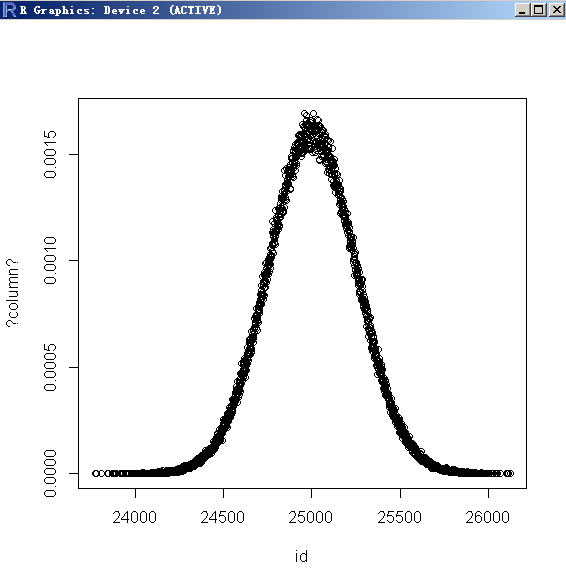postgres=# truncate test;
TRUNCATE TABLE
pg95@db-172-16-3-150-> vi test.sql
\setrandom id 1 50000 gaussian 5.0
insert into test values (:id);

pg95@db-172-16-3-150-> pgbench -M prepared -n -r -f ./test.sql -P 2 -c 16 -j 16 -T 10 --latency-limit=0.2
progress: 2.0 s, 93514.9 tps, lat 0.163 ms stddev 0.119
progress: 4.0 s, 94764.7 tps, lat 0.167 ms stddev 0.117
progress: 6.0 s, 95041.7 tps, lat 0.166 ms stddev 0.112
progress: 8.0 s, 95312.3 tps, lat 0.166 ms stddev 0.112
progress: 10.0 s, 95035.6 tps, lat 0.166 ms stddev 0.112
transaction type: Custom query
scaling factor: 1
query mode: prepared
number of clients: 16
duration: 10 s
number of transactions actually processed: 947354
number of transactions above the 0.2 ms latency limit: 176537 (18.635 %)
latency average: 0.166 ms
latency stddev: 0.114 ms
tps = 94726.249444 (including connections establishing)
tps = 95332.979889 (excluding connections establishing)
statement latencies in milliseconds:
-0.002318       \setrandom id 1 50000 gaussian 5.0
0.160983        insert into test values (:id);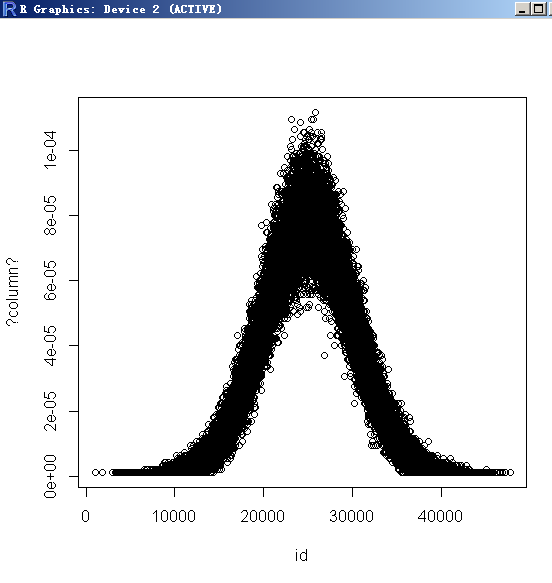### 指数分布例子：

threshold越大，越多的值分布在min附近。
threshold越小，当趋近于0时，接近随机分布，而不是曲线反转。
threshold不能小于0。

postgres=# truncate test;
TRUNCATE TABLE

pg95@db-172-16-3-150-> vi test.sql
\setrandom id 1 50000 exponential 50000
insert into test values (:id);

pg95@db-172-16-3-150-> pgbench -M prepared -n -r -f ./test.sql -P 2 -c 16 -j 16 -T 10
progress: 2.0 s, 94095.8 tps, lat 0.162 ms stddev 0.112
progress: 4.0 s, 95611.9 tps, lat 0.165 ms stddev 0.108
progress: 6.0 s, 94929.7 tps, lat 0.166 ms stddev 0.121
progress: 8.0 s, 96159.4 tps, lat 0.164 ms stddev 0.102
progress: 10.0 s, 95586.2 tps, lat 0.165 ms stddev 0.109
transaction type: Custom query
scaling factor: 1
query mode: prepared
number of clients: 16
duration: 10 s
number of transactions actually processed: 952791
latency average: 0.164 ms
latency stddev: 0.111 ms
tps = 95271.583072 (including connections establishing)
tps = 95879.681335 (excluding connections establishing)
statement latencies in milliseconds:
-0.002375       \setrandom id 1 50000 exponential 1.0
0.160051        insert into test values (:id);

postgres=# analyze test;
ANALYZE

postgres=# select * from pg_stats where attname='id' and tablename='test';
-[ RECORD 1 ]----------+-----------------------------------------------
schemaname             | public
tablename              | test
attname                | id
inherited              | f
null_frac              | 0
avg_width              | 4
n_distinct             | 11
most_common_vals       | {1,2,3,4,5}
most_common_freqs      | {0.632533,0.231967,0.0867,0.0306333,0.0112333}
histogram_bounds       | {6,6,6,6,7,12}
correlation            | 0.458732
most_common_elems      |
most_common_elem_freqs |
elem_count_histogram   |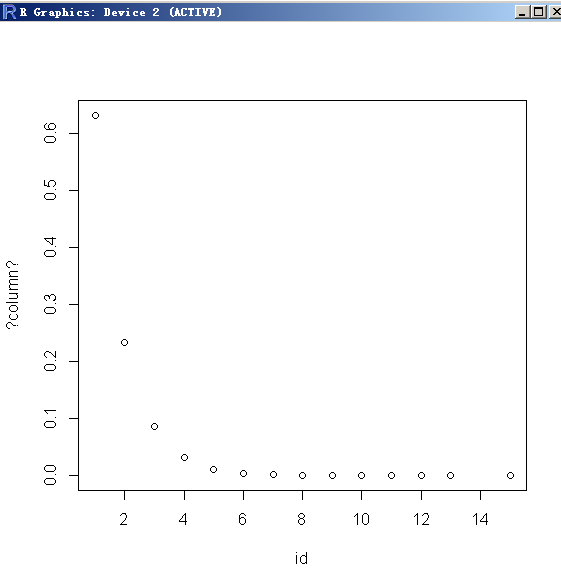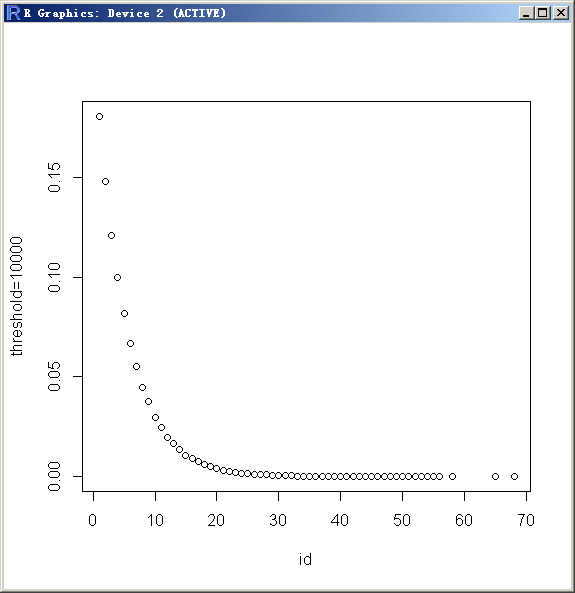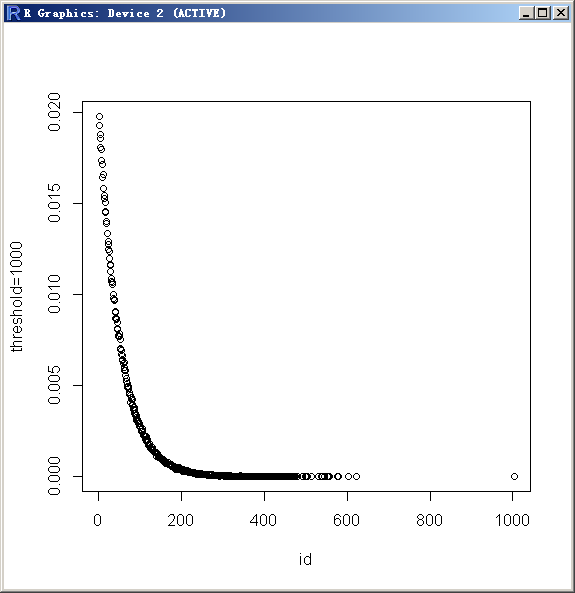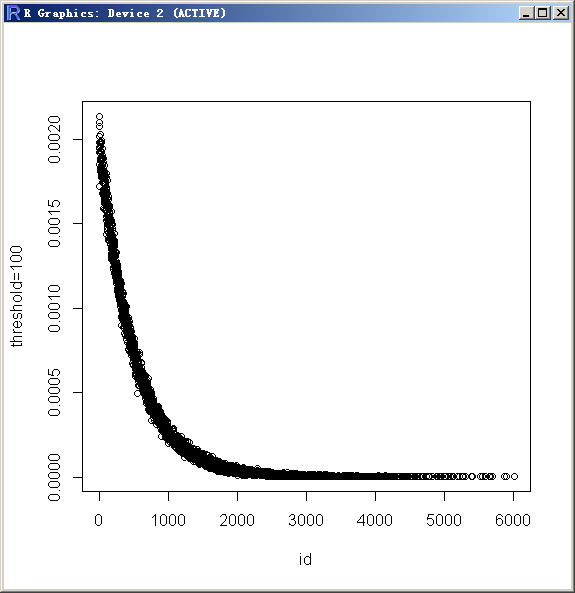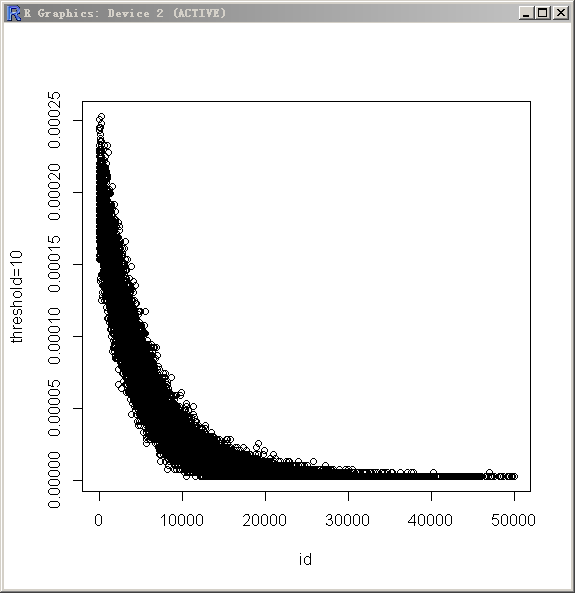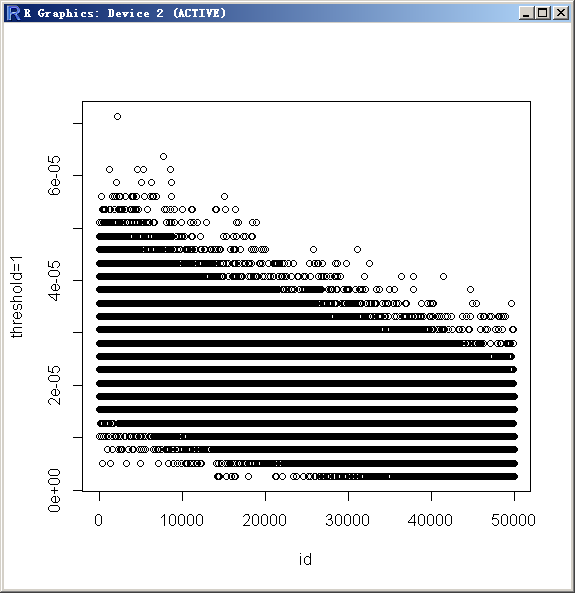threshold=0.000001：已经接近随机分布了。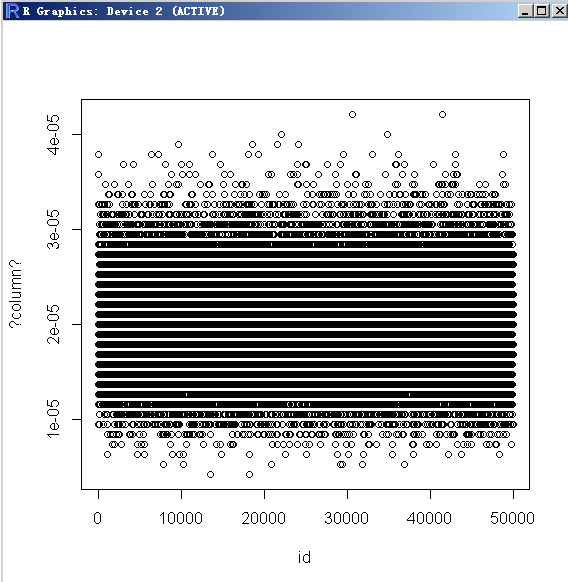[参考]
2. src/bin/pgbench/pgbench.c

/* random number generator: uniform distribution from min to max inclusive */
static int64
getrand(TState *thread, int64 min, int64 max)
{
/*
* Odd coding is so that min and max have approximately the same chance of
* being selected as do numbers between them.
*
* pg_erand48() is thread-safe and concurrent, which is why we use it
* rather than random(), which in glibc is non-reentrant, and therefore
* protected by a mutex, and therefore a bottleneck on machines with many
* CPUs.
*/
return min + (int64) ((max - min + 1) * pg_erand48(thread->random_state));
}

/*
* random number generator: exponential distribution from min to max inclusive.
* the threshold is so that the density of probability for the last cut-off max
* value is exp(-threshold).
*/
static int64
getExponentialRand(TState *thread, int64 min, int64 max, double threshold)
{
double          cut,
uniform,
rand;

Assert(threshold > 0.0);
cut = exp(-threshold);
/* erand in [0, 1), uniform in (0, 1] */

/*
* inner expresion in (cut, 1] (if threshold > 0), rand in [0, 1)
*/
Assert((1.0 - cut) != 0.0);
rand = -log(cut + (1.0 - cut) * uniform) / threshold;
/* return int64 random number within between min and max */
return min + (int64) ((max - min + 1) * rand);
}

/* random number generator: gaussian distribution from min to max inclusive */
static int64
getGaussianRand(TState *thread, int64 min, int64 max, double threshold)
{
double          stdev;
double          rand;

/*
* Get user specified random number from this loop, with -threshold <
* stdev <= threshold
*
* This loop is executed until the number is in the expected range.
*
* As the minimum threshold is 2.0, the probability of looping is low:
* sqrt(-2 ln(r)) <= 2 => r >= e^{-2} ~ 0.135, then when taking the
* average sinus multiplier as 2/pi, we have a 8.6% looping probability in
* the worst case. For a 5.0 threshold value, the looping probability is
* about e^{-5} * 2 / pi ~ 0.43%.
*/
do
{
/*
* pg_erand48 generates [0,1), but for the basic version of the
* Box-Muller transform the two uniformly distributed random numbers
* are expected in (0, 1] (see
* http://en.wikipedia.org/wiki/Box_muller)
*/
double          rand1 = 1.0 - pg_erand48(thread->random_state);
double          rand2 = 1.0 - pg_erand48(thread->random_state);

/* Box-Muller basic form transform */
double          var_sqrt = sqrt(-2.0 * log(rand1));

stdev = var_sqrt * sin(2.0 * M_PI * rand2);

/*
* we may try with cos, but there may be a bias induced if the
* previous value fails the test. To be on the safe side, let us try
* over.
*/
}
while (stdev < -threshold || stdev >= threshold);

/* stdev is in [-threshold, threshold), normalization to [0,1) */
rand = (stdev + threshold) / (threshold * 2.0);

/* return int64 random number within between min and max */
return min + (int64) ((max - min + 1) * rand);
}

/*
* random number generator: generate a value, such that the series of values
* will approximate a Poisson distribution centered on the given value.
*/
static int64
{
/*
* Use inverse transform sampling to generate a value > 0, such that the
* expected (i.e. average) value is the given argument.
*/
double          uniform;

/* erand in [0, 1), uniform in (0, 1] */

return (int64) (-log(uniform) * ((double) center) + 0.5);
}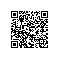使用钉钉扫一扫加入圈子
+ 订阅